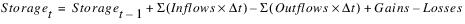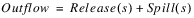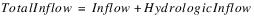Solution Approaches : Simulation : Mathematical Basis for Reservoir Simulation
Mathematical Basis for Reservoir Simulation
Using RiverWare requires knowledge of elementary reservoir modeling mechanics. Reservoir modeling in RiverWare is accomplished using a mass balance approach. The equation for reservoir mass balance is as follows:For this section, it is important to more fully understand the Outflows in the above equation. Outflow in RiverWare, is expressed as follows:Along with the greater detail in the Outflow term of the basic mass balance equation, Total Inflow in RiverWare is expressed as follows:Further refinements may also be made to these equations and the Gain and Loss terms. Some of these include Evaporation, Precipitation, Bank Storage, Pumped Storage Flow, Seepage, Diversion, Return Flow, and Canal Flow.
Given any two of Inflow, Outflow or current Storage, the third variable may be solved for, when the previous Storage is known. Storage can also be determined from the Pool Elevation. Further, the Release (or Energy) and Spill could be specified (together) to determine Outflow.
The discretization of the values in these timeseries is important to recognize. The Inflow and Outflow slots contain the values for the average flow during each timestep, while the Storage and Pool Elevation slots contain the values at the end of each timestep. In other words, the flow values are pulse data, while the elevation and storage values are instantaneous data. Values in RiverWare slots are only as precise as the timestep length used to generate them.
Revised: 01/04/2021